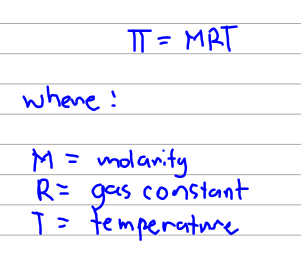# Problem: What is osmotic pressure of a solution of bovine insulin (molar mass, 5700 g mol−1) at 18 °C if 100.0 mL of the solution contains 0.103 g of the insulin?(a) Outline the steps necessary to answer the question.(b) Answer the question.

###### FREE Expert Solution

The osmotic pressure of the solution can be calculated using the formula below:We already have a value for temperature and R is a value that we should memorize so what we are missing is actually the molarity. So we will base our outline on how to get to the molarity first and the even back to intermediate values that would lead to the molarity and eventually the osmotic pressure.

91% (331 ratings)###### Problem Details

What is osmotic pressure of a solution of bovine insulin (molar mass, 5700 g mol−1) at 18 °C if 100.0 mL of the solution contains 0.103 g of the insulin?

(a) Outline the steps necessary to answer the question.